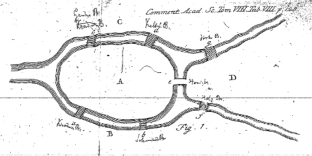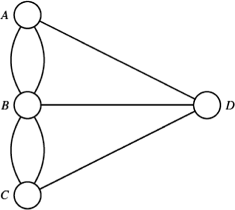# Euler’s Königsberg: the explanatory power of mathematics

## Abstract

The present paper provides an analysis of Euler’s solutions to the Königsberg bridges problem. Euler proposes three different solutions to the problem, addressing their strengths and weaknesses along the way. I put the analysis of Euler’s paper to work in the philosophical discussion on mathematical explanations. I propose that the key ingredient to a good explanation is the degree to which it provides relevant information. Providing relevant information is based on knowledge of the structure in question, graphs in the present case. I also propose computational complexity and logical strength as measures of relevant information.

This is a preview of subscription content, access via your institution.Fig. 1Fig. 2
1. 1.

See Molinini (2012) for a successful example of analyzing Euler’s work in the context of mathematical explanations.

2. 2.

I use the widely available translation (Euler 1956). See Hopkins and Wilson (2004) for a useful overview of Euler’s paper. I thank an anonymous referee for his suggestion to consider Euler’s paper.

3. 3.

Euler does not specify how to carry out the Brute Force Method in detail. One way of implementing it is to write down all (finitely many) paths of length seven starting from one of the areas A,B,C,D, and see whether any one of these paths includes just seven different bridges.

4. 4.

A multigraph is a graph where two vertices can be connected by more than one edge. Consequently, the multiset of edges may contain a pair of vertices more than one time.

5. 5.

Arguably, the fact that Euler’s paper stands at the beginnings of graph theory is its most important innovation. This supports an observation by Rav (1999) that one of the most important roles of proving theorems lies in the novel insights generated by proof methods beyond determining the truth of particular theorems.

6. 6.

Euler does not employ the notation d(X) for the degree of X; his argument hardly uses any algebraic expressions. The reconstruction given here follows Euler closely, but transforms some of his reasoning into algebra in order to make it more accessible.

7. 7.

Euler’s Theorem is different from modern formulations of the theorem in several respects; see, e.g., Diestel (2006, pp. 21) for a modern account. First, Euler asks if it is possible to cross every bridge in Königsberg exactly once, without the assumption that the Euler path should be closed. Second, Euler does not assume that graphs are connected. This is a necessary assumption for the theorem. Third, Euler only proved one direction of the modern version of the theorem, viz. the statement that a closed Euler path exists if, and only if, every area has even number of edges. He did not prove that if a closed Euler path exists, every vertex has an even number of edges; this was only proved 135 years later; see Wilson (1986, p. 270).

8. 8.

The labels IME and SEM are due to Baker (2012); see Mancosu (2011) for a useful overview of the debate on explanations in pure and applied mathematics.

9. 9.

To use an imperfect analogy with the deductive-nomological model of explanation, we need both a general law as well as initial conditions to deduce the explanandum.

10. 10.

Brute Force Methods can be applied whenever a problem is decidable. For example, we may want to find out whether a mathematical structure has a certain property or not, and this can be determined by going through all (finitely many) possible cases. A Brute Force Method then systematically goes through all possible cases, and answers “Yes” if at least one case comes out positive, and “No” otherwise.

11. 11.

We could interpret the Brute Force Method as not being explanatory at all. However, this is, strictly speaking, not true. There are proofs, so-called zero-knowledge proofs, that only show that a result is true, without giving us any knowledge as to why this is so, in a strict, cryptographic sense of knowledge; see Aaronson (2013, Sec. 9.1). However, these are non-classical, probabilistic proofs, and deductive analogues will always convey some information.

12. 12.

This does not speak against all kinds of algorithms that compile lists of paths; the redundancy could be overcome, to a certain degree, by using a clever search algorithm.

13. 13.

Note that while the Intermediate Method and Euler’s Theorm fare better than the Brute Force Method in terms of time complexity, the Brute Force Method is simpler to state than the other methods, i.e., the program-size complexity of the algorithms may increase, and thus be uncorrelated with, explanatory power.

14. 14.

Note that the complexity may depend on the size of both E and V; see, e.g., Gibbons (1985, Ch. 6) for more on issues of complexity.

15. 15.

Note that there may be other fruitful ways of measuring relevant information. To give an example, Mark Colyvan (2012, pp. 83) briefly discusses the idea of using so-called relevant logic to distinguish between explanatory and non-explanatory proofs. I think that this idea is compatible with what I have proposed here.

16. 16.

I thank an anonymous referee for suggesting this perspective of the relation between ontic and epistemic conceptions of explanation.

## References

1. Aaronson, S. (2013). Why philosophers should care about computational complexity. In Copeland, B.J., Posy, C., & Shagrir, O. (Eds.) Computability: Turing, Gödel, Church, and Beyond (pp. 261–328). Cambridge: MIT Press.

2. Baker, A. (2012). Science-driven mathematical explanation. Mind, 121(482), 243–267.

3. Colyvan, M. (2012). An introduction to the philosophy of mathematics. In Cambridge introductions to philosophy. Cambridge: Cambridge University Press.

4. Diestel, R. (2006). Graph theory. graduate texts in mathematics. Berlin: Springer.

5. Euler, L. (1956). The seven bridges of Königsberg. In Newman, J.R. (Ed.) The world of mathematics (Vol. 1, pp. 573–580). New York: Simon and Schuster.

6. Franklin, J. (1994). The formal sciences discover the philosopher’s stone. Studies in History and Philosophy of Science, 25(4), 513–533.

7. Gibbons, A. (1985). Algorithmic graph theory. Cambridge: Cambridge University Press.

8. Gowers, T. (Ed.). (2008). The Princeton Companion to Mathematics. Princeton: Princeton University Press.

9. Hopkins, B., & Wilson, R.J. (2004). The truth about Königsberg. The College Mathematics Journal, 35(3), 198–207.

10. Lange, M. (2013). What makes a scientific explanation distinctively mathematical? British Journal for the Philosophy of Science, 64(3), 485–511.

11. Lange, M. (2014). Aspects of mathematical explanation: symmetry, unity, and salience. Philosophical Review, 123(4), 485–531.

12. Lange, M. (2015). Depth and explanation in mathematics. Philosophia Mathematica, 23(2), 196–214.

13. Lyon, A. (2012). Mathematical explanations of empirical facts, and mathematical realism. Australasian Journal of Philosophy, 90(3), 559–578.

14. Mancosu, P. (2011). Explanation in mathematics, http://plato.stanford.edu/entries/mathematics-explanation/.

15. Mancosu, P., Jorgensen, K.F., & Pedersen, S.A. (Eds.). (2005). Visualization, explanation and reasoning styles in mathematics, synthese library, Vol. 327. Dordrecht: Springer.

16. Molinini, D. (2012). Learning from Euler from mathematical practice to mathematical explanation. Philosophiae Scientiae, 16(1), 105–127.

17. Penco, C. (1994). The philosophy of Michael Dummett, synthese library, Vol. 239, Kluwer Academic Publishers, chap Dummett and Wittgenstein’s Philosophy of Mathematics, pp. 113–136.

18. Pincock, C. (2007). A role for mathematics in the physical sciences. Noûs, 41 (2), 253–275.

19. Pincock, C. (2012). Mathematics and scientific representation. Oxford: Oxford University Press.

20. Pincock, C. (2015). Abstract explanations in science. British Journal for the Philosophy of Science, 66(4), 857–882.

21. Rav, Y. (1999). Why do we prove theorems? Philosophia Mathematica, 7(3), 5–41.

22. Salmon, W.C. (1984). Scientific explanation: three basic conceptions. In PSA: Proceedings of the biennial meeting of the philosophy of science association, vol II: symposia and invited papers (pp. 293–305).

23. Wilholt, T. (2004). Zahl und Wirklichkeit: Eine philosophische Untersuchung über die Anwendbarkeit der Mathematik. Paderborn: Mentis.

24. Wilson, R.J. (1986). An Eulerian trail through Königsberg. Journal of Graph Theory, 10(3), 265–275.

Download references

## Acknowledgements

Thanks to Alan Baker, Claus Beisbart, Matthias Egg, Michael Esfeld, Marion Hämmerli, Marc Lange, Hannes Leitgeb, Philip Mills, Thomas Müller, Christopher Pincock, Antje Rumberg, Tilman Sauer, Raphael Scholl, various anonymous referees, audiences in Bern, Lausanne, and Konstanz for comments on earlier drafts of this paper, to Andreas Verdun for help with the historical literature, to Scott Aaronson for correspondence, and to Dan Ward for proofreading. The usual disclaimer applies.

## Author information

Authors

### Corresponding author

Correspondence to Tim Räz.

## Additional information

This work was partially supported by the Swiss National Science Foundation, grant numbers 100011-124462/1 and 100018-140201/1, as well as by the Templeton World Charity Foundation through grant TWCF0078/AB46

## Rights and permissions

Reprints and Permissions